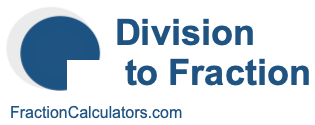Division as a Fraction CalculatorWelcome to the Division as a Fraction Calculator. This clever calculator can calculate and convert your division problem to a fraction.

There is a strong correlation between a division problem and a fraction, and we will show you exactly how they relate. Please enter your division problem below to get started.

÷

Here are some division as a fraction conversions that you may find interesting: